# Problems implementing multiple range sideband fit (unbinned extended maximum likelihood) for a 2d data set

Hello,

I am trying to do an unbinned extended maximum likelihood fit into multiple sidebands on a 2-dimensional data-set.
I have followed following Roofit tutorials:
1> https://root.cern/doc/v610/rf312__multirangefit_8C.html

The relevant part of the code is :

Fit variables are : M_dz and deltam

Part I: (setting the sideband range)

``````  //------------mdz left side-band----------//
M_dz.setRange("sbml",1.81,1.85);
deltam.setRange("sbml",0.14,0.15);

//------------mdz left side-band----------//

M_dz.setRange("sbmr",1.88,1.92);
deltam.setRange("sbmr",0.14,0.15);

//-----fitting---------//
// to extend pdf RooAddPdf is used as suggested in https://root.cern/doc/v610/rf312__multirangefit_8C.html
RooFitResult *rs1 =mdzdel1.fitTo(*ds1,Range("sbml,sbmr"),Extended(kTRUE), Minos(0),Hesse(0), NumCPU(4),Save(),Timer(kTRUE));``

//-------plotting-------//
RooPlot *mdz_frame = M_dz.frame();
ds1->plotOn(mdz_frame);
mdzdel1.plotOn(mdz_frame, Range("sbml,sbmr"),NormRange("sbml,sbmr"),LineColor(kBlue), LineStyle(kSolid));
mdzdel1.paramOn(mdz_frame);

const double nData = ds1->sumEntries(" ", "sbmr,sbml");

RooPlot *deltam_frame = deltam.frame();
ds1->plotOn(deltam_frame);
mdzdel1.plotOn(deltam_frame, Range("sbml,sbmr"),NormRange("sbml,sbmr"), LineColor(kBlue), LineStyle(kSolid),Normalization(nData, RooAbsReal::NumEvent));
``````

The normalization(yield) obtained from fit doesn’t look right and so is the fit projection for variable deltam (see attached document deltam.pdf or fig. below)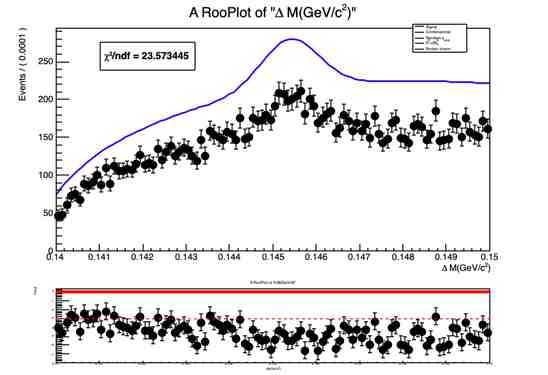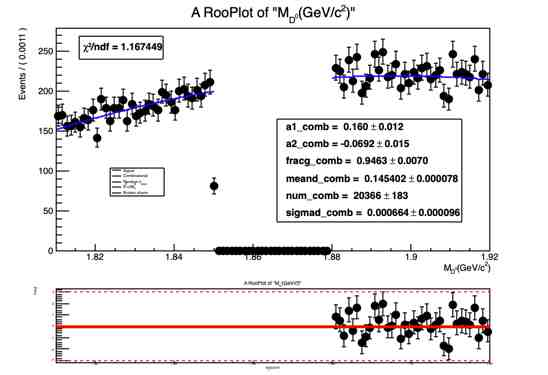I have found a similar problem reported here :

I have implemented the suggested solution but it’s not working.
I do not get any warning regarding the normalization of fit projection. (see attached snapshots

)

I am attaching the fit macro part4_all1.C part4_all1.C (23.1 KB) ,
logfile sb_fit.txt (101.7 KB)
deltam fit projection deltam.pdf (33.3 KB)
M_dz fit projection Mdz.pdf (27.7 KB)!

Hi @amroo,

I suspect you need a `ProjectionRange`, see here:
RooAbsPdf::plotOn()
and here:
https://root.cern.ch/doc/master/rf311__rangeplot_8C.html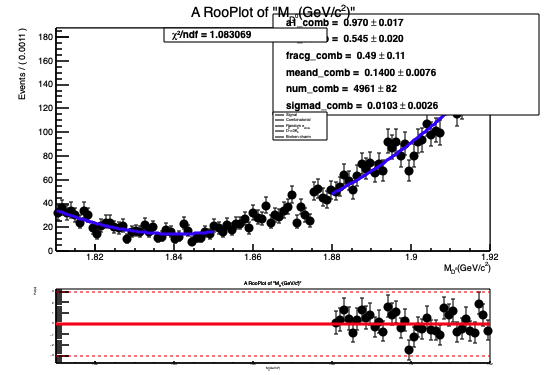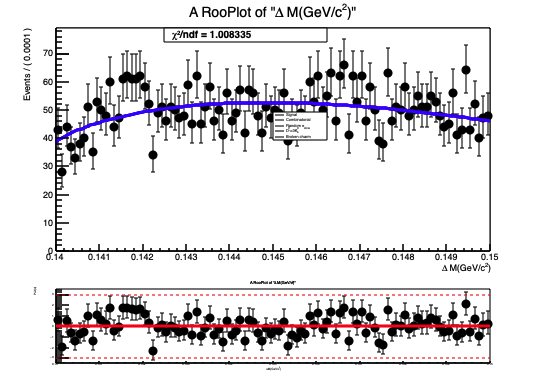Two more things:

• You can enclose code between three ` to make it typeset nicely in the forum.
• Your macro was quite useful, but either include the data or include `myPdf->generate(...)` to generate some toy data, so we can run it immediately.

Hi @StephanH,

Thanks for reply and posting related suggestions.
I am attaching my dataset signal_new.txt (327.5 KB)
I have found a bunch of questions to clarify :

1> I have found that If I use RooAddPdf to extend my pdf as suggested here :

i.e.

``````RooAddPdf mdzdel1("mdzdel1","mdzdel1",mdzdelm_comb,num_comb) ;
RooFitResult *rs1 =mdzdel1.fitTo(*ds1,Range("sbml,sbmr"),Extended(kTRUE), Minos(0),Hesse(0), NumCPU(4),Save(),Timer(kTRUE));
``````

I get the wrong yield returned from the fitter (see the value of num_comb). Fit Results :

`RooExtendPdf mdzdel1("mdzdel1","mdzdel1",mdzdelm_comb,num_comb,"sbml,sbmr") ;`
I get the correct yields from the fit. See the value of num_comb = 14580

It seems using RooExtendPdf with a specified range is the correct solution ?? contrary to what is suggested here: https://root.cern.ch/doc/master/rf204a__extrangefit__RooAddPdf_8C.html.

2> Coming to the issue with fit projections when doing sideband fit in multiple ranges.
(for plots in this part I am using RooExtend with range to extend my pdf)
a> Range(“sbml,sbmr”): To draw fit projections in the specific range.
b> NormRange(“sbml,sbmr”) : To normalize the pdf in only the specified range.

You are suggesting to use : ProjectionRange() such that when plotting for deltam it integrates over only specified Mdz range.
If I do :
a>

``````mdzdel1.plotOn(deltam_frame,Range("sbml,sbmr"),ProjectionRange("sbml,sbmr"),NormRange("sbml,sbmr"), LineColor(kBlue), LineStyle(kSolid),Normalization(nData, RooAbsReal::NumEvent));
``````

I get the following projection in deltam: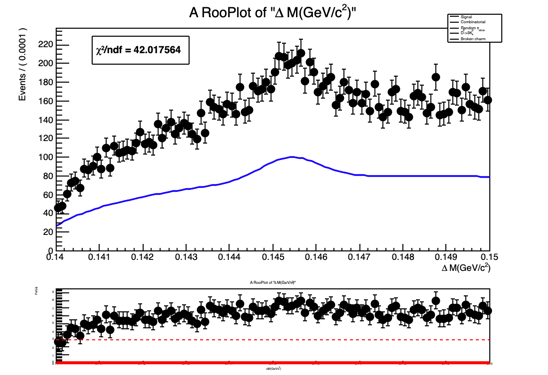b> If I remove the " Normalization(nData, RooAbsReal::NumEvent));"

``````mdzdel1.plotOn(deltam_frame,Range("sbml,sbmr"),ProjectionRange("sbmlp,sbmrp"),NormRange("sbml,sbmr"), LineColor(kBlue), LineStyle(kSolid));
``````

Then I get following correct projection in deltam: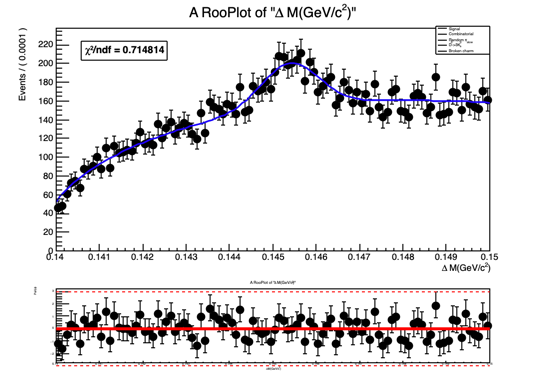Just one final question:
For M_dz projection, I am getting pull distribution for only a part of the range
This is what I am doing :

``````RooPlot *pull1_frame = M_dz.frame();
RooHist* hpull1 = mdz_frame->pullHist(); //mdz_frame is where pdf and data are plotted
``````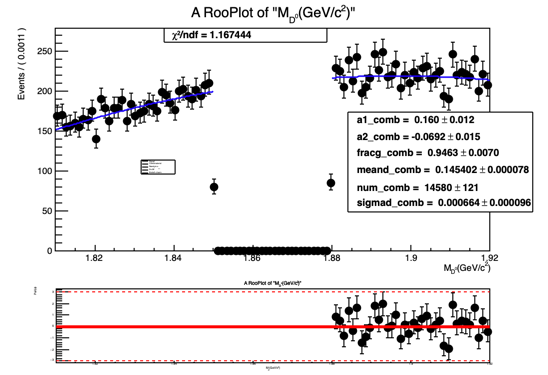Any Ideas on how to fix this ?
Thanks a lot for all your time.
Aman

I guess it depends on the range where you measure `num_comb`. When using the RooAddPdf, you can change this range using “SumCoefRange” found in the documentation here.

Yes, this is likely because `nData` that you get and the number of events that’s actually plotted are different. But defining the range of the projection integrals worked now. That’s nice.

Ok, that’s funny. This is likely because the two fit ranges are plotted separately. What RooFit is doing to create the pulls is here:
https://root.cern.ch/doc/master/RooPlot_8cxx_source.html#l01136

You could retrieve the curve and data that’s on the left and right, and merge them using the addBin() and addBinWithError() functions below. Then, you use residHist to create the residual or pull plot.

This topic was automatically closed 14 days after the last reply. New replies are no longer allowed.